•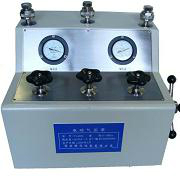电动气压源XY-4 `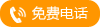` `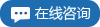`
•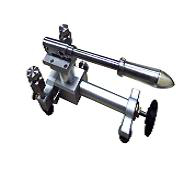便携式手动气压 `` ``
•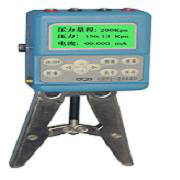智能便携式数字 `` ``
•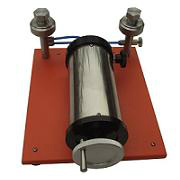手动微压源XY-2 `` ``
•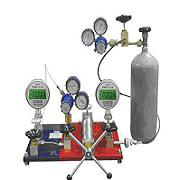减压器校验仪XY `` ``
•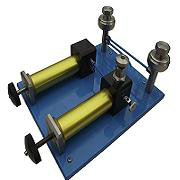气体压力源XY-2 `` ``
•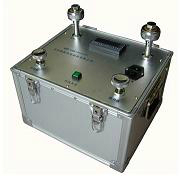电动真空源XY20 `` ``
•电动液压源 `` ``
•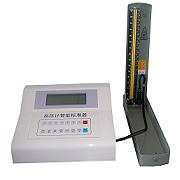血压计校验仪 `` ``
•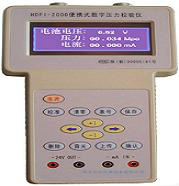智能数字压力校 `` ``
•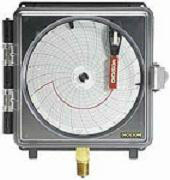智能压力天平 `` ``
•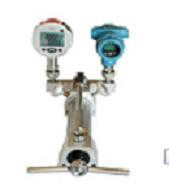智能压力校验仪 `` ``
•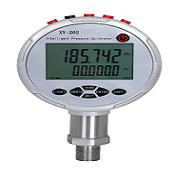智能压力校验仪 `` ``
•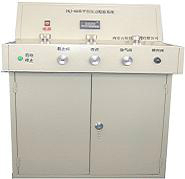批量快速压力校 `` ``
•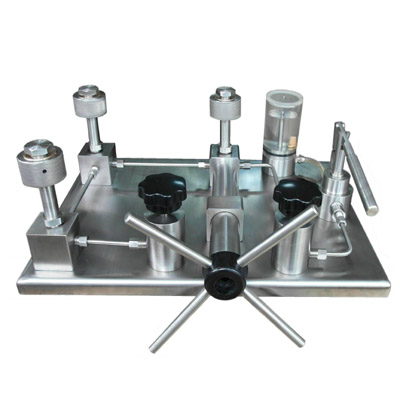高压水介质压力 `` ``
•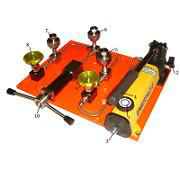高压液体压力源 `` ``
•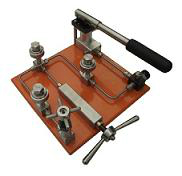高压气体压力源 `` ``
•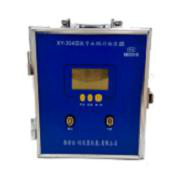数字血压计校准 `` ``
•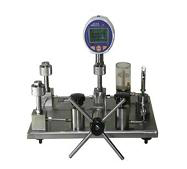通用型压力源FL `` ``
•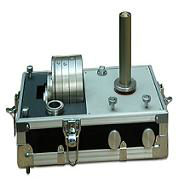浮球压力计 `` ``